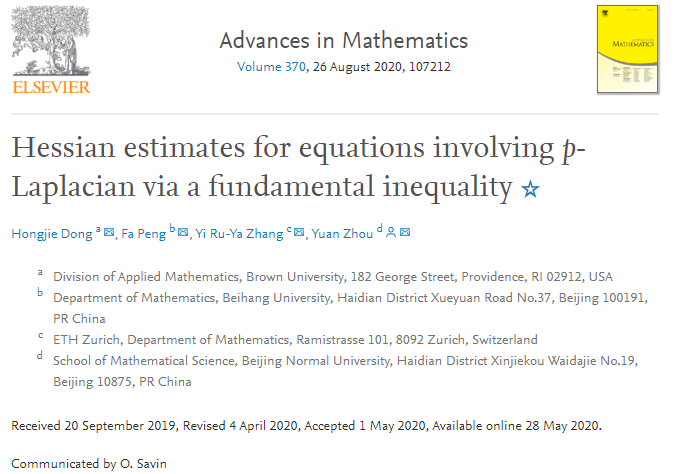﻿ Research Findings of School of Mathematical Sciences Published in Advances in Mathematics-BEIHANG UNIVERSITY
• ###### Global
SearchNews & Events
2020Research Findings of School of Mathematical Sciences Published in Advances in Mathematics
Release time：September 29, 2020 / Feng Yueyue

On September 24, an article entitled “Hessian estimates for equations involving p-Laplacian via a fundamental inequality” by Fa Peng, a Ph.D student from the School of Mathematical Sciences, his supervisor Prof. Yuan Zhou and two other collaborators was published in Advances in Mathematics. The article illustrated researchers’ latest breakthrough in the field of nonlinear degradation equation.In the article, the researchers revealed a basic inequality in high dimension which will apply the equation established by Koch and others on the plane in high dimension space, and for the first time applied to \$p\$- harmonic operator, fully answering the public question put forward by Lindqvist and others. In addition, due to the high universality of the inequality for operator equations containing \$p\$-Laplace, some problems (such as \$p\$-harmonic equation, \$p\$-Laplace equation with singular term, parabolic \$p\$-Laplace equation, non-homogeneous \$P \$-Laplace type equation) can be solved directly by using this inequality. By using the approximation viewpoint, the structure of the equation and the inequality to calculate and solve the equation, researchers can obtain unprecedented results.

Prof. Hongjie Dong from Brown University and Prof. Yi Ru-Ya Zhang from Swiss Federal Institute of Technology Zurich also participated in the research work.

https://www.sciencedirect.com/science/article/pii/S0001870820302383

Reported by Li Tiantian

Reviewed by Yuan Xing

Edited by Jia Aiping

Translated by Feng Yueyue

Recommended News
March 1, 2022
February 7, 2022
January 17, 2022
January 12, 2022
###### Calendar